# Introduction to Ratio

Introduction to Ratio• We are sharing 35 blocks of chocolate in the ratio 4:3.
• There are two numbers in this ratio, so we are sharing the chocolate between two people.
• One person gets 4 parts for every 3 parts that the other person gets.
• We break off groups of 4 blocks and groups of 3 blocks until there is nothing left.
• The total on the left is 20 blocks and the total on the right is 15 blocks.
• 20 + 15 = 35, which is the total amount of blocks that we started with.
The numbers in a ratio tell us how to share our total amount.• There are 16 blocks in total that will be shared between two people in the ratio 1:3.
• This means that every time we give the person on the left 1 block we give the person on the right 3 blocks.
• We can share the chocolate in this proportion until it is all gone.
• The person on the left has 4 blocks and the person on the right has 12 blocks.
• We write the answer as a ratio of 4:12.
• We can check our answer since 4 + 12 = 16, which is the total amount we started with.Supporting Lessons# Introduction to Sharing in a Ratio

A ratio tells us how a total amount has been broken up or can be broken up. In this lesson, we will be sharing a total amount between people. The amount of numbers in the ratio tell us how many people we will be sharing between.

We use a colon ‘:’ to separate the numbers in a ratio and this is a way to help us recognise that we have a ratio rather than a list of numbers.

In the image below, we can see an example of a ratio with two numbers: ‘4’ and ‘3’. Because there are two numbers, we will be sharing the amount between two people.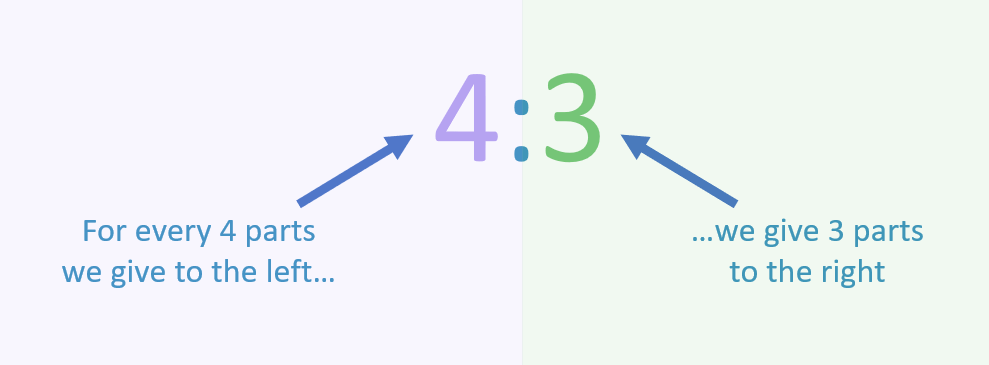The numbers of ‘4’ and ‘3’ tell us the proportions (or relative sizes) of how we will share the amount.

This ratio means that for every 4 parts we give to the person on the left, we give 3 parts to the person on the right.In our first example of sharing in a ratio above, we have a bar of chocolate made up of 35 blocks in total.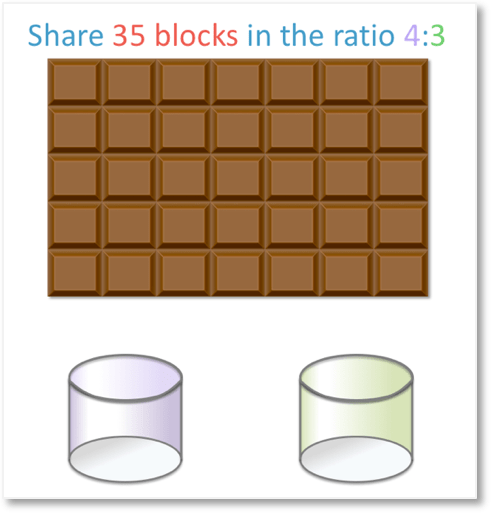We are asked to share the total amount of 35 blocks in the ratio 4:3.

This means that for every 4 parts one person is given, another person is given 3 parts.

In this introduction to ratio, we imagine that we place the blocks for the first person in a purple pot, and the blocks for the second person in a green pot.For every 4 blocks of chocolate we place in the purple pot, we place 3 blocks in the green pot.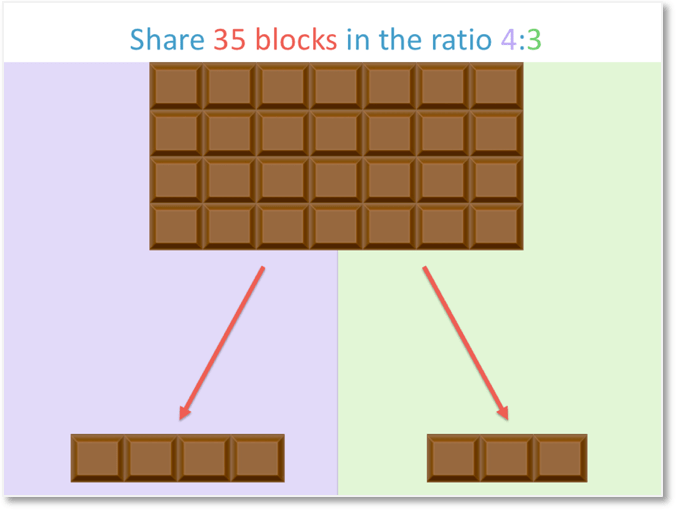We can share this ratio by breaking off 4 parts and placing them in the purple pot and then breaking off 3 parts and placing them in the green pot.

Every time we move 4 blocks over to the left, we must move 3 blocks over to the right.

This will ensure that we keep the proportion of 4 parts to 3 parts.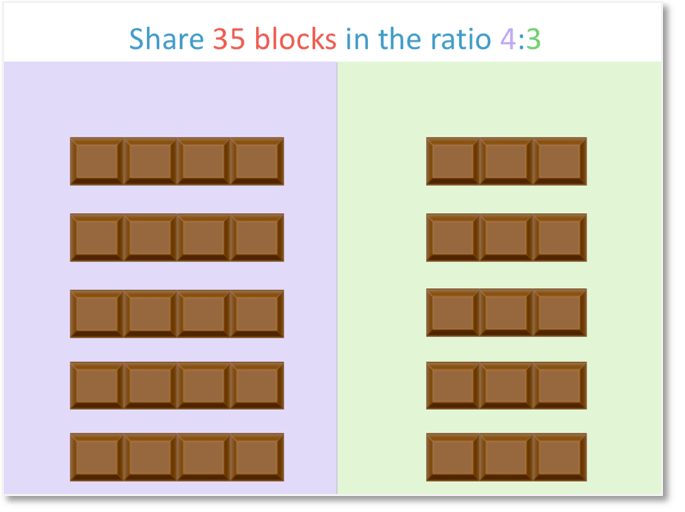We continue until we have shared all of the chocolate.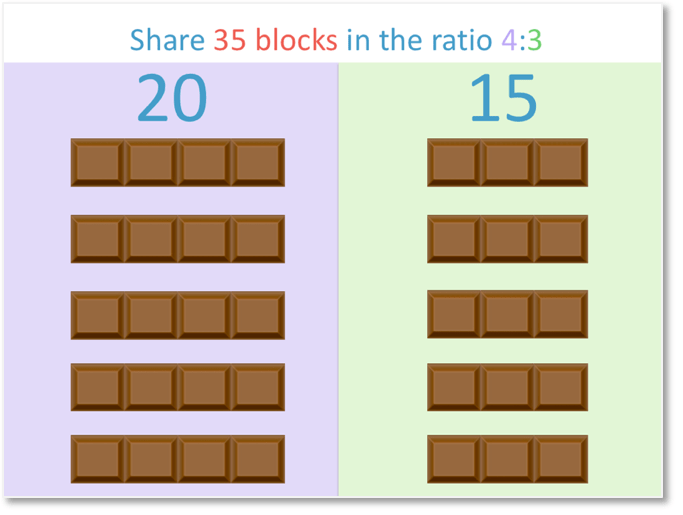If we count the blocks of chocolate, we can see that the first person receives 20 blocks and the second person receives 15 blocks.This gives us a ratio of 20:15.

We can check our answer since 20:15 simplifies to 4:3, which was our original ratio that we were asked to share in. We simply divide 20 by 5 to get 4 and divide 15 by 5 to get 3.

An equivalent ratio is a multiple of another ratio. All numbers in the ratio have been multiplied by the same number.

The ratio of 20:15 is an equivalent ratio to 4:3 because both numbers have been multiplied by 5.

We also see that 20 + 15 = 35, which was the original total amount of chocolate blocks before we shared them in a ratio.

We have introduced ratio by sharing this amount physically, moving 4 blocks and 3 blocks at a time.

When teaching ratio for the first time, it is recommended that you use a physical set of items that can be shared by moving the parts, such as chocolate or counters.

Above there are some sharing in a ratio worksheets, which you can cut into parts using scissors.Now try our lesson on Sharing in a Ratio using the Bar Model where we learn how to share an amount in a ratio using the bar model.